# My Lecture Notes from Linear Algebra

22 Apr 2016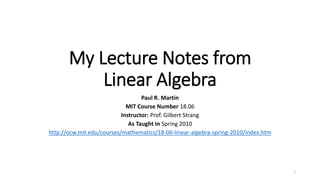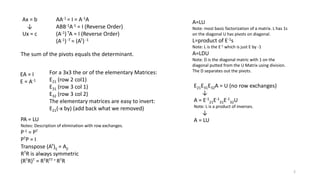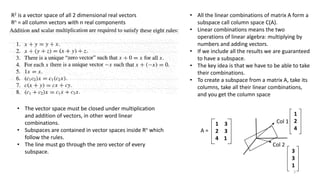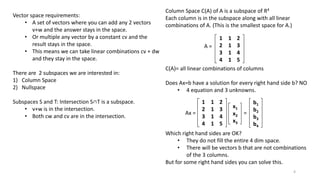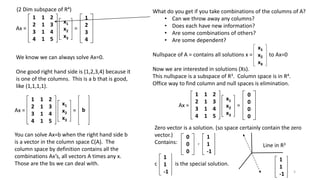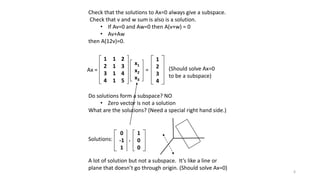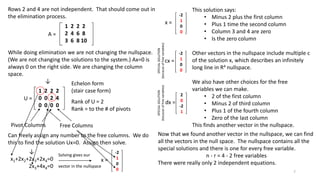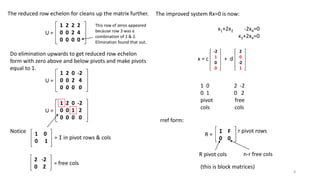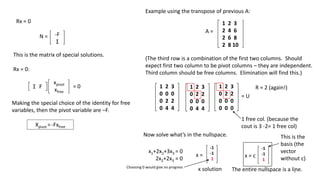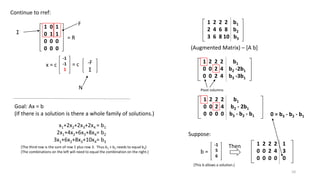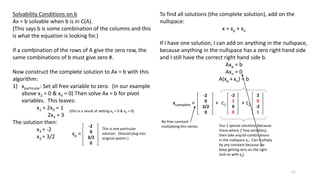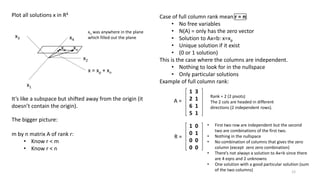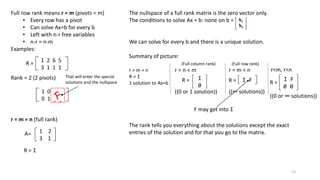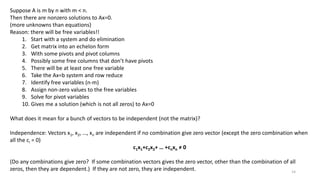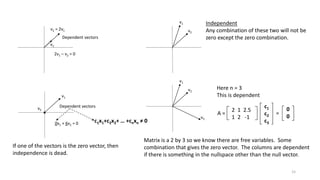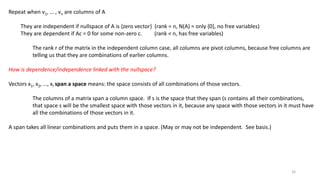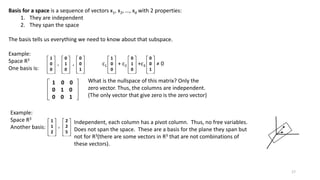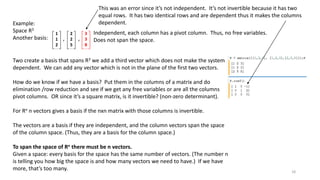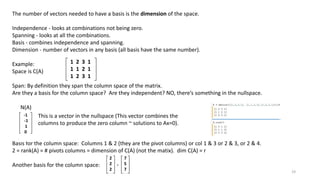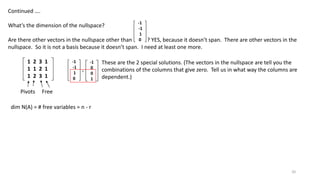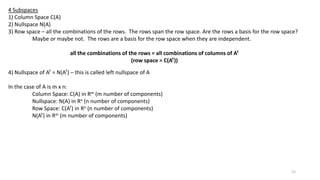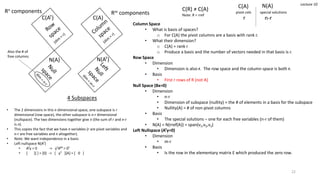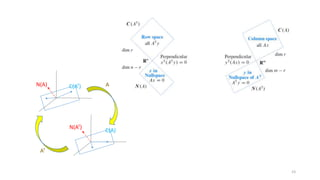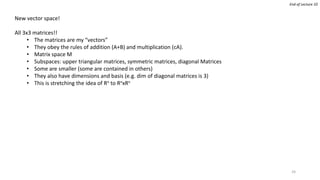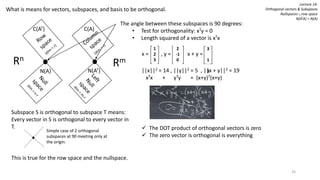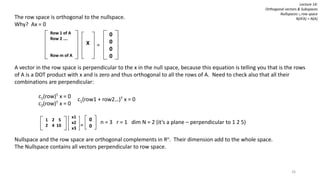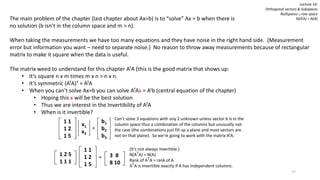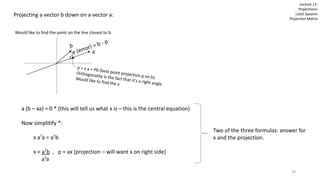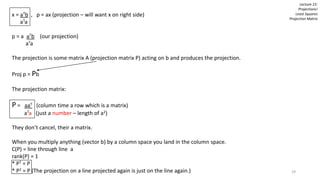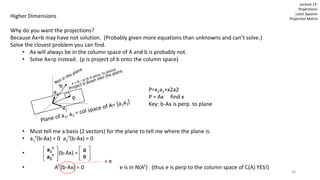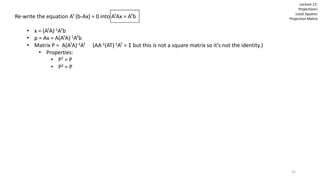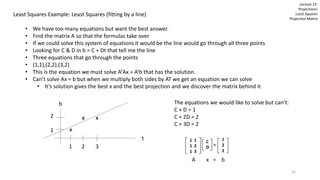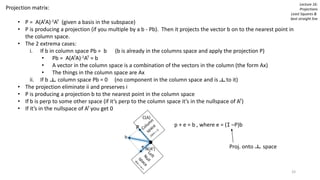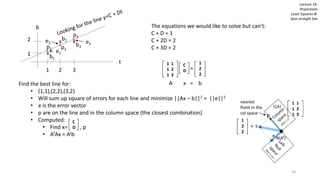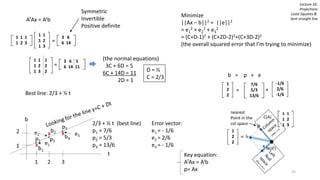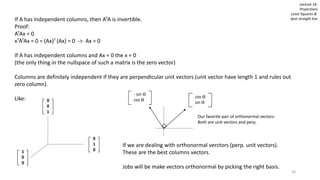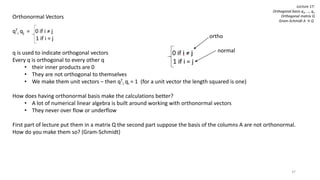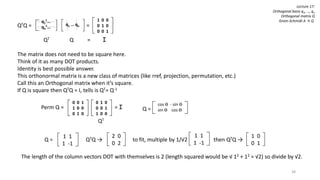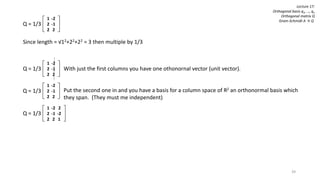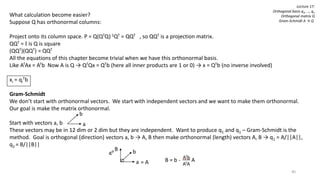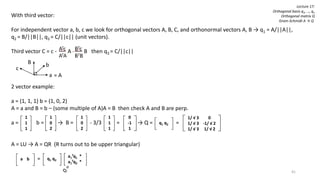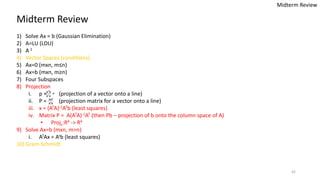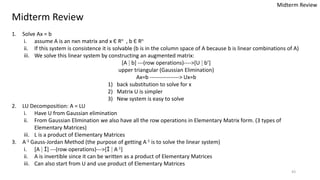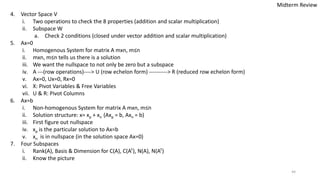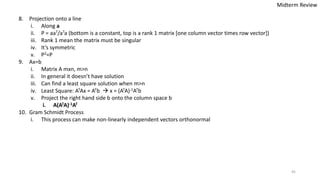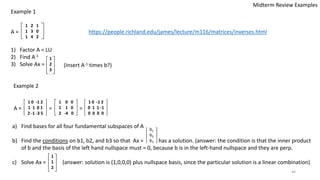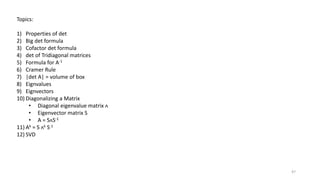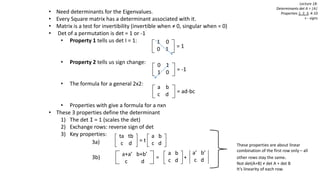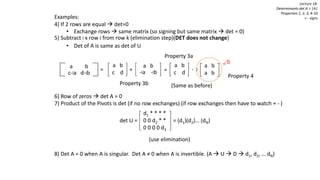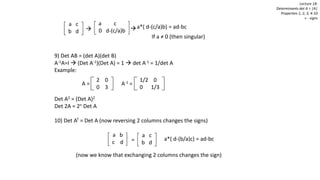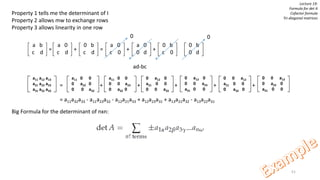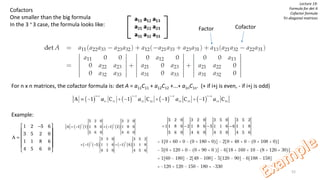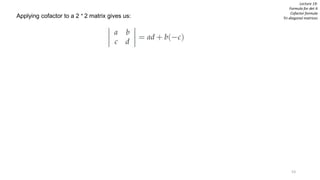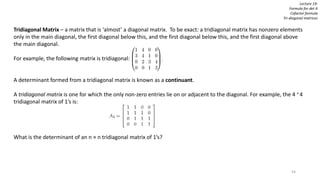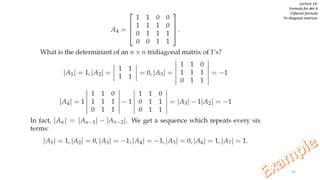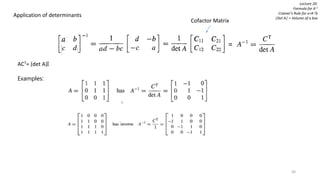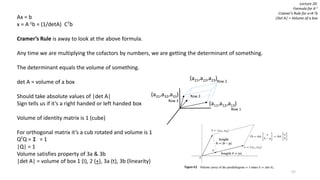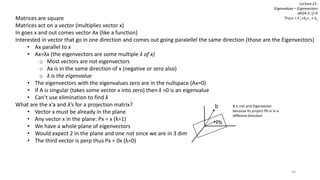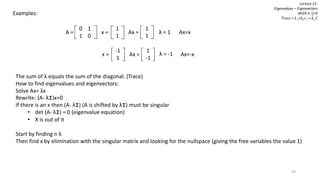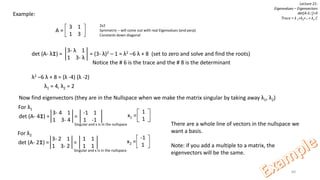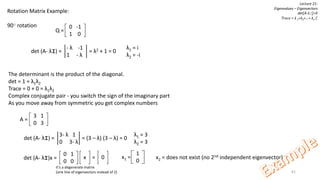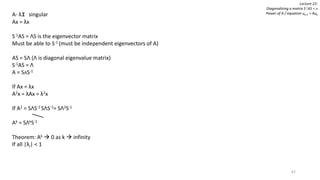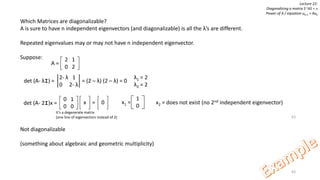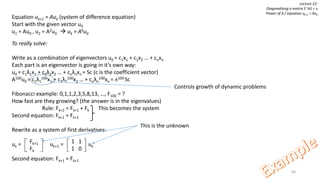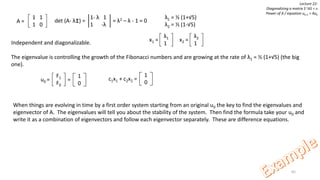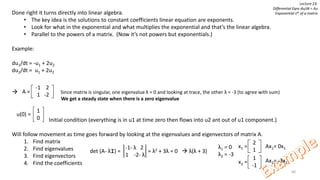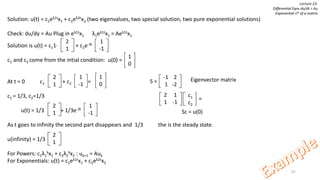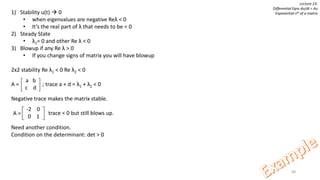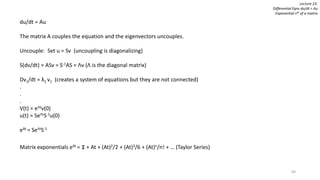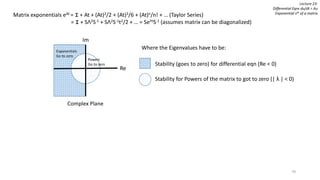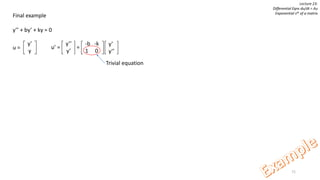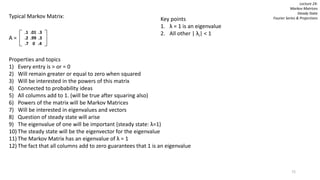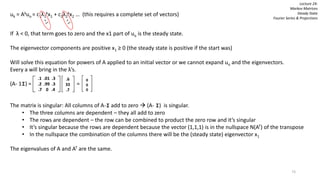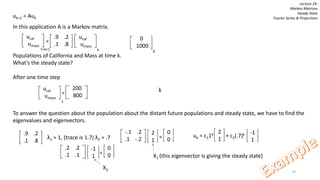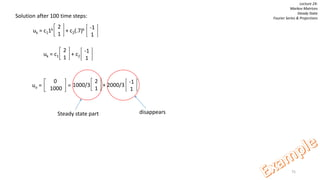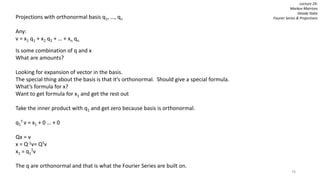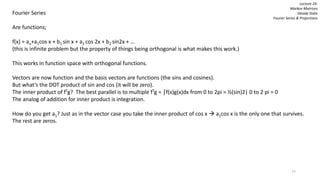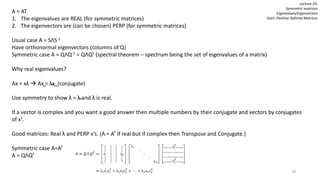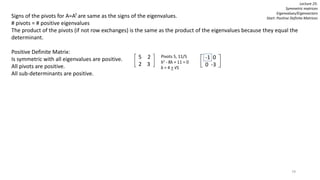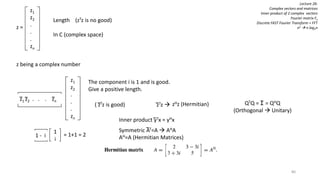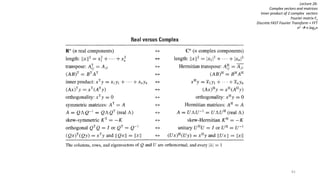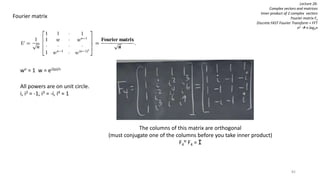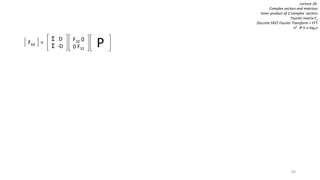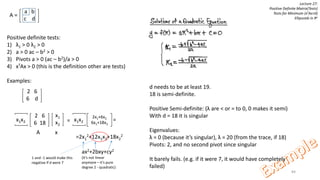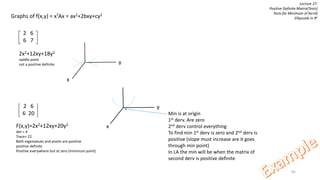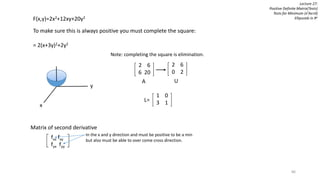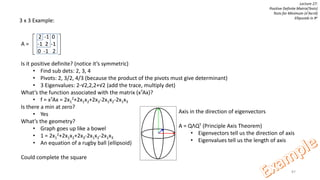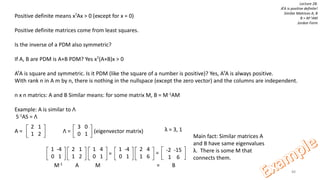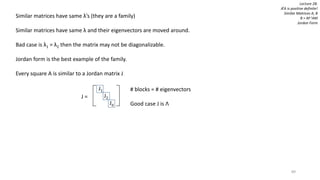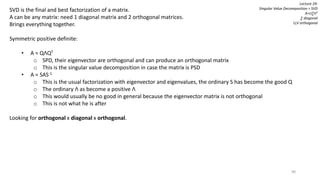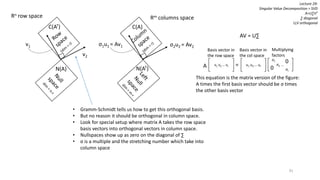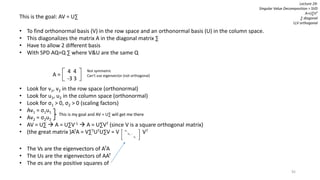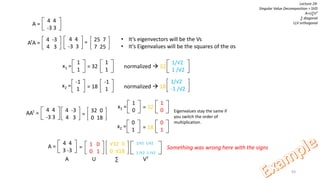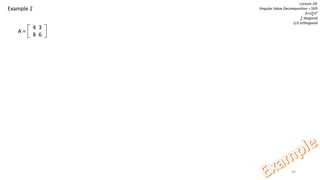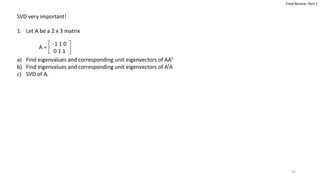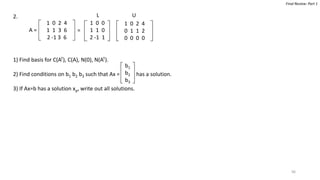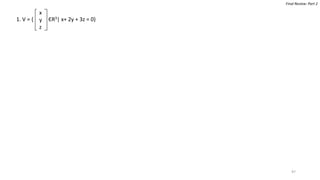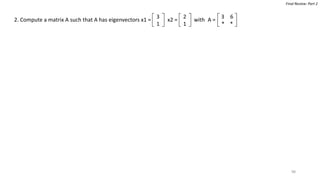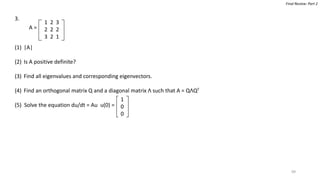1 sur 99

### My Lecture Notes from Linear Algebra

• 1. My Lecture Notes from Linear Algebra Paul R. Martin MIT Course Number 18.06 Instructor: Prof. Gilbert Strang As Taught In Spring 2010 http://ocw.mit.edu/courses/mathematics/18-06-linear-algebra-spring-2010/index.htm 1
• 2. Ax = b ↓ Ux = c AA-1 = I = A-1A ABB-1A-1 = I (Reverse Order) (A-1) TA = I (Reverse Order) (A-1) -T = (AT) -1 A=LU Note: most basic factorization of a matrix. L has 1s on the diagonal U has pivots on diagonal. L=product of E-1s Note: L is the E-1 which is just E by -1 A=LDU Note: D is the diagonal matric with 1 on the diagonal putted from the U Matrix using division. The D separates out the pivots. The sum of the pivots equals the determinant. EA = I E = A-1 For a 3x3 the or of the elementary Matrices: E21 (row 2 col1) E31 (row 3 col 1) E32 (row 3 col 2) The elementary matrices are easy to invert: E21(-x by) (add back what we removed) E21E31E32A = U (no row exchanges) ↓ A = E-1 21E-1 31E-1 32U Note: L is a product of inverses. ↓ A = LUPA = LU Notes: Description of elimination with row exchanges. P-1 = PT PTP = I Transpose (AT)ij = Aji RTR is always symmetric (RTR)T = RTRTT = RTR 2
• 3. R2 is a vector space of all 2 dimensional real vectors Rn = all column vectors with n real components • The vector space must be closed under multiplication and addition of vectors, in other word linear combinations. • Subspaces are contained in vector spaces inside Rn which follow the rules. • The line must go through the zero vector of every subspace. • All the linear combinations of matrix A form a subspace call column space C(A). • Linear combinations means the two operations of linear algebra: multiplying by numbers and adding vectors. • If we include all the results we are guaranteed to have a subspace. • The key idea is that we have to be able to take their combinations. • To create a subspace from a matrix A, take its columns, take all their linear combinations, and you get the column space A = 1 3 2 3 4 1 Col 1 Col 2 1 2 4 3 3 1 3
• 4. Column Space C(A) of A is a subspace of R4 Each column is in the subspace along with all linear combinations of A. (This is the smallest space for A.) C(A)= all linear combinations of columns Does Ax=b have a solution for every right hand side b? NO • 4 equation and 3 unknowns. Which right hand sides are OK? • They do not fill the entire 4 dim space. • There will be vectors b that are not combinations of the 3 columns. But for some right hand sides you can solve this. Vector space requirements: • A set of vectors where you can add any 2 vectors v+w and the answer stays in the space. • Or multiple any vector by a constant cv and the result stays in the space. • This means we can take linear combinations cv + dw and they stay in the space. There are 2 subspaces we are interested in: 1) Column Space 2) Nullspace Subspaces S and T: Intersection S∩T is a subspace. • v+w is in the intersection. • Both cw and cv are in the intersection. A = 1 1 2 2 1 3 3 1 4 4 1 5 Ax = 1 1 2 2 1 3 3 1 4 4 1 5 x1 x2 x3 = b1 b2 b3 b4 4
• 5. We know we can always solve Ax=0. One good right hand side is (1,2,3,4) because it is one of the columns. This is a b that is good, like (1,1,1,1). Ax = 1 1 2 2 1 3 3 1 4 4 1 5 x1 x2 x3 = 1 2 3 4 Ax = 1 1 2 2 1 3 3 1 4 4 1 5 x1 x2 x3 = b You can solve Ax=b when the right hand side b is a vector in the column space C(A). The column space by definition contains all the combinations Ax’s, all vectors A times any x. Those are the bs we can deal with. What do you get if you take combinations of the columns of A? • Can we throw away any columns? • Does each have new information? • Are some combinations of others? • Are some dependent? Nullspace of A = contains all solutions x = to Ax=0 Now we are interested in solutions (Xs). This nullspace is a subspace of R3. Column space is in R4. Office way to find column and null spaces is elimination. (2 Dim subspace of R4) Ax = 1 1 2 2 1 3 3 1 4 4 1 5 x1 x2 x3 = 0 0 0 0 Zero vector is a solution. (so space certainly contain the zero vector.) Contains: , c is the special solution. 0 0 0 1 1 -1 1 1 -1 1 1 -1 Line in R3 5
• 6. Check that the solutions to Ax=0 always give a subspace. Check that v and w sum is also is a solution. • If Av=0 and Aw=0 then A(v+w) = 0 • Av+Aw then A(12v)=0. Ax = 1 1 2 2 1 3 3 1 4 4 1 5 x1 x2 x3 = 1 2 3 4 Do solutions form a subspace? NO • Zero vector is not a solution What are the solutions? (Need a special right hand side.) Solutions: , A lot of solution but not a subspace. It’s like a line or plane that doesn’t go through origin. (Should solve Ax=0) 0 -1 1 1 0 0 (Should solve Ax=0 to be a subspace) 6
• 7. Rows 2 and 4 are not independent. That should come out in the elimination process. While doing elimination we are not changing the nullspace. (We are not changing the solutions to the system.) Ax=0 is always 0 on the right side. We are changing the column space. ↓ A = 1 2 2 2 2 4 6 8 3 6 8 10 U = 1 2 2 2 0 0 2 4 0 0 0 0 Echelon form (stair case form) Rank of U = 2 Rank = to the # of pivots Pivot Columns Free Columns Can freely assign any number to the free columns. We do this to find the solution Ux=0. Assign then solve. -2 1 0 0 x = ↓ x1+2x2+2x3+2x4=0 2x3+4x4=0 Solving gives our vector in the nullspace -2 1 0 0 x = This solution says: • Minus 2 plus the first column • Plus 1 time the second column • Column 3 and 4 are zero • Is the zero column Other vectors in the nullspace include multiple c of the solution x, which describes an infinitely long line in R4 nullspace. We also have other choices for the free variables we can make. • 2 of the first column • Minus 2 of third column • Plus 1 of the fourth column • Zero of the last column This finds another vector in the nullspace. -2 1 0 0 cx = 2 0 -2 1 dx = SPECIALSOLUTION (becauseoffreevariables) SPECIALSOLUTION (becauseoffreevariables) Now that we found another vector in the nullspace, we can find all the vectors in the null space. The nullspace contains all the special solutions and there is one for every free variable. n - r = 4 - 2 free variables There were really only 2 independent equations. 7
• 8. The reduced row echelon for cleans up the matrix further. U = 1 2 2 2 0 0 2 4 0 0 0 0 This row of zeros appeared because row 3 was a combination of 1 & 2. Elimination found that out. Do elimination upwards to get reduced row echelon form with zero above and below pivots and make pivots equal to 1. U = 1 2 0 -2 0 0 2 4 0 0 0 0 U = 1 2 0 -2 0 0 1 2 0 0 0 0 Notice 1 0 0 1 = I in pivot rows & cols 2 -2 0 2 = free cols -2 1 0 0 x = c 2 0 -2 1 + d x1+2x2 -2x4=0 x3+2x4=0 The improved system Rx=0 is now: 1 0 2 -2 0 1 0 2 pivot free cols cols rref form: I F 0 0 R = r pivot rows R pivot cols n-r free cols (this is block matrices) 8
• 9. Rx = 0 N = -F I This is the matrix of special solutions. Rx = 0: I F xpivot xfree = 0 Xpivot = -Fxfree Making the special choice of the identity for free variables, then the pivot variable are –F. A = 1 2 3 2 4 6 2 6 8 2 8 10 Example using the transpose of previous A: (The third row is a combination of the first two columns. Should expect first two column to be pivot columns – they are independent. Third column should be free columns. Elimination will find this.) 1 2 3 0 0 0 0 2 2 0 4 4 1 2 3 0 2 2 0 0 0 0 4 4 1 2 3 0 2 2 0 0 0 0 0 0 R = 2 (again!) = U 1 free col. (because the cout is 3 -2= 1 free col) Now solve what’s in the nullspace. -1 -1 1 x = x1+2x2+3x3 = 0 2x2+2x3 = 0 x solution -1 -1 1 x = c The entire nullspace is a line. This is the basis (the vector without c) Choosing 0 would give no progress 9
• 10. Continue to rref: 1 0 1 0 1 1 0 0 0 0 0 0 = R I F -1 -1 1 x = c = c -F I N Goal: Ax = b (If there is a solution is there a whole family of solutions.) x1+2x2+2x3+2x4 = b1 2x1+4x2+6x3+8x4= b2 3x1+6x2+8x3+10x4= b3 (The third row is the sum of row 1 plus row 3. Thus b1 + b2 needs to equal b3) (The combinations on the left will need to equal the combination on the right.) 1 2 2 2 b1 2 4 6 8 b2 3 6 8 10 b3 (Augmented Matrix) – [A b] 1 2 2 2 b1 0 0 2 4 b2 -2b1 0 0 2 4 b3 -3b1 Pivot columns 1 2 2 2 b1 0 0 2 4 b2 - 2b1 0 0 0 0 b3 - b2 - b1 0 = b3 - b2 - b1 1 2 2 2 1 0 0 2 4 3 0 0 0 0 0 -1 5 6 b = Suppose: Then (This b allows a solution.) 10
• 11. Solvability Conditions on b Ax = b solvable when b is in C(A). (This says b is some combination of the columns and this is what the equation is looking for.) If a combination of the rows of A give the zero row, the same combinations of b must give zero #. Now construct the complete solution to Ax = b with this algorithm: 1) xparticular: Set all free variable to zero. (in our example above x2 = 0 & x4 = 0) Then solve Ax = b for pivot variables. This leaves: x1 + 2x3 = 1 2x3 = 3 The solution then: x3 = -2 x3 = 3/2 (this is a result of setting x2 = 0 & x4 = 0) -2 0 3/2 0 xp = This is one particular solution. (Should plug into original system.) To find all solutions (the complete solution), add on the nullspace: x = xp + xn If I have one solution, I can add on anything in the nullspace, because anything in the nullspace has a zero right hand side and I still have the correct right hand side b. Axp = b Axn = 0 A(xp + xn) = b -2 0 3/2 0 xcomplete = + -2 1 0 0 c1 2 0 -2 1 + c2 Our 2 special solutions (because there where 2 free variables), then take any/all combinations in the nullspace xn. Can multiply by any constant because we keep getting zero on the right (not so with xp). No free constant multiplying this vector. 11
• 12. Plot all solutions x in R4 x2 x1 x3 x4 It’s like a subspace but shifted away from the origin (it doesn’t contain the origin). xp xn x = xp + xn xn was anywhere in the plane which filled out the plane The bigger picture: m by n matrix A of rank r: • Know r < m • Know r < n Case of full column rank mean r = n: • No free variables • N(A) = only has the zero vector • Solution to Ax=b: x=xp • Unique solution if it exist • (0 or 1 solution) This is the case where the columns are independent. • Nothing to look for in the nullspace • Only particular solutions Example of full column rank: 1 3 2 1 6 1 5 1 A = Rank = 2 (2 pivots) The 2 cols are headed in different directions (2 independent rows). 1 0 0 1 0 0 0 0 R = • First two row are independent but the second two are combinations of the first two. • Nothing in the nullspace • No combination of columns that gives the zero column (except zero zero combination) • There’s not always a solution to Ax=b since there are 4 eqns and 2 unknowns • One solution with a good particular solution (sum of the two columns) 12
• 13. Full row rank means r = m (pivots = m) • Every row has a pivot • Can solve Ax=b for every b • Left with n-r free variables • n-r = n-m Examples: R = Rank = 2 (2 pivots) 1 2 6 5 3 1 1 1 1 0 _ _ 0 1 _ _ That will enter the special solutions and the nullspace r = m = n (full rank) A= 1 2 3 1 R = I The nullspace of a full rank matrix is the zero vector only. The conditions to solve Ax = b: none on b = We can solve for every b and there is a unique solution. Summary of picture: r = m = n R = I 1 solution to Ax=b b1 b2 r = n < m ((0 or 1 solution)) r = m < n ((∞ solutions)) R = I 0 I FR = (Full row rank)(Full column rank) F may get into I r<m, r<n I F 0 0 R = ((0 or ∞ solutions)) The rank tells you everything about the solutions except the exact entries of the solution and for that you go to the matrix. 13
• 14. Suppose A is m by n with m < n. Then there are nonzero solutions to Ax=0. (more unknowns than equations) Reason: there will be free variables!! 1. Start with a system and do elimination 2. Get matrix into an echelon form 3. With some pivots and pivot columns 4. Possibly some free columns that don’t have pivots 5. There will be at least one free variable 6. Take the Ax=b system and row reduce 7. Identify free variables (n-m) 8. Assign non-zero values to the free variables 9. Solve for pivot variables 10. Gives me a solution (which is not all zeros) to Ax=0 What does it mean for a bunch of vectors to be independent (not the matrix)? Independence: Vectors x1, x2, …, xn are independent if no combination give zero vector (except the zero combination when all the ci = 0) c1x1+c2x2+ … +cnxn ≠ 0 (Do any combinations give zero? If some combination vectors gives the zero vector, other than the combination of all zeros, then they are dependent.) If they are not zero, they are independent. 14
• 15. Dependent vectors v2 = 2v1 v1 2v1 – v2 = 0 v1 v2 Dependent vectors 0v1 + 6v2 = 0 If one of the vectors is the zero vector, then independence is dead. c1x1+c2x2+ … +cnxn ≠ 0 v1 v2 Independent Any combination of these two will not be zero except the zero combination. v1 v2 v3 Here n = 3 This is dependent 2 1 2.5 1 2 -1 A = c1 c2 c3 0 0 = Matrix is a 2 by 3 so we know there are free variables. Some combination that gives the zero vector. The columns are dependent if there is something in the nullspace other than the null vector. 15
• 16. Repeat when v1, … , vn are columns of A They are independent if nullspace of A is {zero vector} (rank = n, N(A) = only {0}, no free variables) They are dependent if Ac = 0 for some non-zero c. (rank < n, has free variables) The rank r of the matrix in the independent column case, all columns are pivot columns, because free columns are telling us that they are combinations of earlier columns. How is dependence/independence linked with the nullspace? Vectors x1, x2, …, xl span a space means: the space consists of all combinations of those vectors. The columns of a matrix span a column space. If s is the space that they span (s contains all their combinations, that space s will be the smallest space with those vectors in it, because any space with those vectors in it must have all the combinations of those vectors in it. A span takes all linear combinations and puts them in a space. (May or may not be independent. See basis.) 16
• 17. Basis for a space is a sequence of vectors x1, x2, …, xd with 2 properties: 1. They are independent 2. They span the space The basis tells us everything we need to know about that subspace. Example: Space R3 One basis is: 1 0 0 0 1 0 0 0 1‘ ‘ 1 0 0 0 1 0 0 0 1 c1 + c2 +c3 ≠ 0 1 0 0 0 1 0 0 0 1 What is the nullspace of this matrix? Only the zero vector. Thus, the columns are independent. (The only vector that give zero is the zero vector) 1 1 2 2 2 5 ‘ Example: Space R3 Another basis: Independent, each column has a pivot column. Thus, no free variables. Does not span the space. These are a basis for the plane they span but not for R3(there are some vectors in R3 that are not combinations of these vectors). 17
• 18. 1 1 2 2 2 5 3 3 8‘ ‘ Independent, each column has a pivot column. Thus, no free variables. Does not span the space. Example: Space R3 Another basis: Two create a basis that spans R3 we add a third vector which does not make the system dependent. We can add any vector which is not in the plane of the first two vectors. How do we know if we have a basis? Put them in the columns of a matrix and do elimination /row reduction and see if we get any free variables or are all the columns pivot columns. OR since it’s a square matrix, is it invertible? (non-zero determinant). For Rn n vectors gives a basis if the nxn matrix with those columns is invertible. The vectors are a basis if they are independent, and the column vectors span the space of the column space. (Thus, they are a basis for the column space.) To span the space of Rn there must be n vectors. Given a space: every basis for the space has the same number of vectors. (The number n is telling you how big the space is and how many vectors we need to have.) If we have more, that’s too many. This was an error since it’s not independent. It’s not invertible because it has two equal rows. It has two identical rows and are dependent thus it makes the columns dependent. 18
• 19. The number of vectors needed to have a basis is the dimension of the space. Independence - looks at combinations not being zero. Spanning - looks at all the combinations. Basis - combines independence and spanning. Dimension - number of vectors in any basis (all basis have the same number). Example: Space is C(A) Span: By definition they span the column space of the matrix. Are they a basis for the column space? Are they independent? NO, there’s something in the nullspace. N(A) Basis for the column space: Columns 1 & 2 (they are the pivot columns) or col 1 & 3 or 2 & 3, or 2 & 4. 2 = rank(A) = # pivots columns = dimension of C(A) (not the matix). dim C(A) = r Another basis for the column space: 1 2 3 1 1 1 2 1 1 2 3 1 -1 -1 1 0 This is a vector in the nullspace (This vector combines the columns to produce the zero column ~ solutions to Ax=0). 2 2 2 7 5 7 ‘ 19
• 20. Continued …. What’s the dimension of the nullspace? Are there other vectors in the nullspace other than ? YES, because it doesn’t span. There are other vectors in the nullspace. So it is not a basis because it doesn’t span. I need at least one more. 1 2 3 1 1 1 2 1 1 2 3 1 -1 -1 1 0 -1 -1 1 0 -1 0 0 1 These are the 2 special solutions. (The vectors in the nullspace are tell you the combinations of the columns that give zero. Tell us in what way the columns are dependent.) Pivots Free , dim N(A) = # free variables = n - r 20
• 21. 4 Subspaces 1) Column Space C(A) 2) Nullspace N(A) 3) Row space – all the combinations of the rows. The rows span the row space. Are the rows a basis for the row space? Maybe or maybe not. The rows are a basis for the row space when they are independent. all the combinations of the rows = all combinations of columns of AT (row space = C(AT)) 4) Nullspace of AT = N(AT) – this is called left nullspace of A In the case of A is m x n: Column Space: C(A) in Rm (m number of components) Nullspace: N(A) in Rn (n number of components) Row Space: C(AT) in Rn (n number of components) N(AT) in Rm (m number of components) 21
• 22. 4 Subspaces Rm componentsRn components Column Space • What is basis of spaces? o For C(A) the pivot columns are a basis with rank r. • What their dimension? o C(A) = rank r o Produce a basis and the number of vectors needed in that basis is r. Row Space • Dimension • Dimension is also r. The row space and the column space is both r. • Basis • First r rows of R (not A) Null Space (Bx=0) • Dimension • n-r • Dimension of subspace (nullity) = the # of elements in a basis for the subspace • Nullity(A) = # of non-pivot columns • Basis • The special solutions – one for each free variables (n-r of them) • N(A) = N(rref(A)) = span(v1,v2,v3) Left Nullspace (ATy=0) • Dimension • m-r • Basis • Is the row in the elementary matrix E which produced the zero row. Lecture 10 C(AT) C(A) N(A) C(A) N(A) pivot cols special solutions n-rr • The 2 dimensions in this n dimensional space, one subspace is r dimensional (row space), the other subspace is n-r dimensional (nullspace). The two dimensions together give n (the sum of r and n-r is n). • This copies the fact that we have n variables (r are pivot variables and n-r are free variables and n altogether). • Note: We want independence in a basis • Left nullspace N(AT) • ATy = 0 -> yTATT = 0T • [ ][ ] =  -> [ yT ][A] = [ 0 ] N(AT) C(R) ≠ C(A) Note: R = rref 22 Also the # of free columns
• 24. New vector space! All 3x3 matrices!! • The matrices are my “vectors” • They obey the rules of addition (A+B) and multiplication (cA). • Matrix space M • Subspaces: upper triangular matrices, symmetric matrices, diagonal Matrices • Some are smaller (some are contained in others) • They also have dimensions and basis (e.g. dim of diagonal matrices is 3) • This is stretching the idea of Rn to RnxRn End of Lecture 10 24
• 25. Lecture 14: Orthogonal vectors & Subspaces Nullspaces ∟row space N(ATA) = N(A) What is means for vectors, subspaces, and basis to be orthogonal. C(AT) C(A) N(A) N(AT) The angle between these subspaces is 90 degrees: • Test for orthogonality: xTy = 0 • Length squared of a vector is xTx 1 2 3 2 -1 0 x = , y = x + y = 3 1 3||x||2 = 14 , ||y||2 = 5 , ||x + y||2 = 19 xTx + yTy = (x+y)T(x+y)  The DOT product of orthogonal vectors is zero  The zero vector is orthogonal is everything Subspace S is orthogonal to subspace T means: Every vector in S is orthogonal to every vector in T. Simple case of 2 orthogonal subspaces at 90 meeting only at the origin. This is true for the row space and the nullspace. RmRn 25
• 26. The row space is orthogonal to the nullspace. Why? Ax = 0 A vector in the row space is perpendicular to the x in the null space, because this equation is telling you that is the rows of A is a DOT product with x and is zero and thus orthogonal to all the rows of A. Need to check also that all their combinations are perpendicular: c1(row)T x = 0 c2(row)T x = 0 Nullspace and the row space are orthogonal complements in Rn. Their dimension add to the whole space. The Nullspace contains all vectors perpendicular to row space. Row 1 of A Row 2 …. Row m of A X = 0 0 0 0 c1(row1 + row2…)T x = 0 1 2 5 2 4 10 x1 x2 x3 = 0 0 n = 3 r = 1 dim N = 2 (it’s a plane – perpendicular to 1 2 5) Lecture 14: Orthogonal vectors & Subspaces Nullspaces ∟row space N(ATA) = N(A) 26
• 27. The main problem of the chapter (last chapter about Ax=b) is to “solve” Ax = b when there is no solution (b isn’t in the column space and m > n). When taking the measurements we have too many equations and they have noise in the right hand side. (Measurement error but information you want – need to separate noise.) No reason to throw away measurements because of rectangular matrix to make it square when the data is useful. The matrix weed to understand for this chapter ATA (this is the good matrix that shows up: • It’s square n x m times m x n > n x n. • It’s symmetric (ATA)T = ATA • When you can’t solve Ax=b you can solve ATAx = Atb (central equation of the chapter) • Hoping this x will be the best solution • Thus we are interest in the Invertibility of ATA • When is it invertible? 1 1 1 2 1 5 b1 b2 b3 x1 x2 = Can’t solve 3 equations with ony 2 unknown unless vector b is in the column space thus a combination of the columns but unusually not the case (the combinations just fill up a plane and most vectors are not on that plane). So we’re going to work with the matrix ATA. 1 1 1 2 1 5 1 2 5 1 1 1 = 3 8 8 10 (It’s not always invertible.) N(ATA) = N(A) Rank of ATA = rank of A ATA is invertible exactly if A has independent columns. Lecture 14: Orthogonal vectors & Subspaces Nullspaces ∟row space N(ATA) = N(A) 27
• 28. Lecture 15: Projections! Least Squares Projection Matrix Projecting a vector b down on a vector a: Would like to find the point on the line closest to b. b a a (b – xa) = 0 * (this will tell us what x is – this is the central equation) Now simplitify *: x aTa = aTb x = aTb , p = ax (projection – will want x on right side) aTa Two of the three formulas: answer for x and the projection. 28
• 29. x = aTb , p = ax (projection – will want x on right side) aTa p = a aTb (our projection) aTa The projection is some matrix A (projection matrix P) acting on b and produces the projection. Proj p = Pb The projection matrix: P = aaT (column time a row which is a matrix) aTa (just a number – length of a2) They don’t cancel, their a matrix. When you multiply anything (vector b) by a column space you land in the column space. C(P) = line through line a rank(P) = 1 * PT = P * P2 = P (The projection on a line projected again is just on the line again.) Lecture 15: Projections! Least Squares Projection Matrix 29
• 30. Lecture 15: Projections! Least Squares Projection Matrix Higher Dimensions Why do you want the projections? Because Ax=b may have not solution. (Probably given more equations than unknowns and can’t solve.) Solve the closest problem you can find. • Ax will always be in the column space of A and b is probably not. • Solve Ax=p instead. (p is project of b onto the column space) • Must tell me a basis (2 vectors) for the plane to tell me where the plane is. • a1 T(b-Ax) = 0 a2 T(b-Ax) = 0 • (b-Ax) = • AT (b-Ax) = 0 e is in N(AT) (thus e is perp to the column space of C(A) YES!) a1 a2 P=x1a1+x2a2 P = Ax find x Key: b-Ax is perp. to plane a1 T a2 T 0 0 30
• 31. Re-write the equation AT (b-Ax) = 0 into ATAx = ATb • x = (ATA)-1ATb • p = Ax = A(ATA)-1ATb • Matrix P = A(ATA)-1AT (AA-1(AT)-1AT = I but this is not a square matrix so it’s not the identity.) • Properties: • PT = P • P2 = P Lecture 15: Projections! Least Squares Projection Matrix 31
• 32. Lecture 15: Projections! Least Squares Projection Matrix Least Squares Example: Least Squares (fitting by a line) • We have too many equations but want the best answer. • Find the matrix A so that the formulas take over • If we could solve this system of equations it would be the line would go through all three points • Looking for C & D in b = C + Dt that tell me the line • Three equations that go through the points • (1,1),(2,2),(3,2) • This is the equation we must solve ATAx = Atb that has the solution. • Can’t solve Ax = b but when we multiply both sides by AT we get an equation we can solve • It’s solution gives the best x and the best projection and we discover the matrix behind it t b x x x 1 2 3 2 1 The equations we would like to solve but can’t: C + D = 1 C + 2D = 2 C + 3D = 2 1 1 1 2 1 3 C D = 1 2 2 A x = b 32
• 33. Lecture 16: Projections Least Squares & best straight line Projection matrix: • P = A(ATA)-1AT (given a basis in the subspace) • P is producing a projection (if you multiple by a b - Pb). Then it projects the vector b on to the nearest point in the column space. • The 2 extrema cases: i. If b in column space Pb = b (b is already in the columns space and apply the projection P) • Pb = A(ATA)-1AT = b • A vector in the column space is a combination of the vectors in the column (the form Ax) • The things in the column space are Ax ii. If b column space Pb = 0 (no component in the column space and is to it) • The projection eliminate ii and preserves i • P is producing a projection b to the nearest point in the column space • If b is perp to some other space (if it’s perp to the column space it’s in the nullspace of AT) • If it’s in the nullspace of AT you get 0 b e P p + e = b , where e = (I –P)b Proj. onto space 33
• 34. b1 Lecture 16: Projections Least Squares & best straight line t b x x x 1 2 3 2 1 The equations we would like to solve but can’t: C + D = 1 C + 2D = 2 C + 3D = 2 1 1 1 2 1 3 C D = 1 2 2 A x = bFind the best line for: • (1,1),(2,2),(3,2) • Will sum up square of errors for each line and minimize ||Ax – b||2 = ||e||2 • e is the error vector • p are on the line and in the column space (the closest combination) • Computed: • Find x= , p • ATAx = Atb e1 e2 e3 b2 b3p2 p1 p3 C D 1 2 2 = nearest Point in the col space 34
• 35. Lecture 16: Projections Least Squares & best straight line 1 1 1 2 1 3 1 1 1 1 2 3 3 6 6 14= ATAx = Atb Symmetric Invertible Positive definite 3 6 5 6 14 11= 1 1 1 1 2 2 1 3 2 (the normal equations) 3C + 6D = 5 6C + 14D = 11 2D = 1 Minimize ||Ax – b||2 = ||e||2 = e1 2 + e2 2 + e3 2 = (C+D-1)2 + (C+2D-2)2+(C+3D-2)2 (the overall squared error that I’m trying to minimize) D = ½ C = 2/3 Best line: 2/3 + ½ t 2/3 + ½ t (best line) p1 = 7/6 p2 = 5/3 p3 = 13/6 Error vector: e1 = - 1/6 e2 = 2/6 e3 = - 1/6 b = p + e 1 2 2 7/6 5/3 13/6 = + -1/6 2/6 -1/6 1 2 2 = nearest Point in the col space 35 Key equation: ATAx = Atb p= Ax
• 36. 36 Lecture 16: Projections Least Squares & best straight lineIf A has independent columns, then ATA is invertible. Proof: ATAx = 0 xTATAx = 0 = (Ax)T (Ax) = 0 -> Ax = 0 If A has independent columns and Ax = 0 the x = 0 (the only thing in the nullspace of such a matrix is the zero vector) Columns are definitely independent if they are perpendicular unit vectors (unit vector have length 1 and rules out zero column). Like: 1 0 0 0 1 0 0 0 1 If we are dealing with orthonormal verctors (perp. unit vectors). These are the best columns vectors. Jobs will be make vectors orthonormal by picking the right basis. cos ϴ sin ϴ - sin ϴ cos ϴ Our favorite pair of orthonormal vectors: Both are unit vectors and perp.
• 37. 37 Lecture 17: Orthogonal basis q1, …, qn Orthogonal matrix Q Gram-Schmidt A → Q Orthonormal Vectors qT i qj = 0 if i ≠ j 1 if i = j q is used to indicate orthogonal vectors Every q is orthogonal to every other q • their inner products are 0 • They are not orthogonal to themselves • We make them unit vectors – then qT i qi = 1 (for a unit vector the length squared is one) How does having orthonormal basis make the calculations better? • A lot of numerical linear algebra is built around working with orthonormal vectors • They never over flow or underflow First part of lecture put them in a matrix Q the second part suppose the basis of the columns A are not orthonormal. How do you make them so? (Gram-Schmidt) ortho normal
• 38. 38 Lecture 17: Orthogonal basis q1, …, qn Orthogonal matrix Q Gram-Schmidt A → Q QTQ = = QT Q = I The matrix does not need to be square here. Think of it as many DOT products. Identity is best possible answer. This orthonormal matrix is a new class of matrices (like rref, projection, permutation, etc.) Call this an Orthogonal matrix when it’s square. If Q is square then QTQ = I, tells is QT= Q-1 q1 T… qn T… q1 … qn c 1 0 0 0 1 0 0 0 1 cos ϴ - sin ϴ sin ϴ cos ϴc 0 1 0 0 0 1 1 0 0 c 0 0 1 1 0 0 0 1 0 Perm Q = = I QT Q = 1 1 1 -1 Q = QTQ → to fit, multiple by 1/√2 then QTQ → 2 0 0 2 1 1 1 -1 1 0 0 1 The length of the column vectors DOT with themselves is 2 (length squared would be √ 12 + 12 = √2) so divide by √2.
• 39. 39 Lecture 17: Orthogonal basis q1, …, qn Orthogonal matrix Q Gram-Schmidt A → Q 1 -2 2 -1 2 2 Q = 1/3 Since length = √12+22+22 = 3 then multiple by 1/3 1 -2 2 -1 2 2 Q = 1/3 With just the first columns you have one othonornal vector (unit vector). Put the second one in and you have a basis for a column space of R2 an orthonormal basis which they span. (They must me independent) 1 -2 2 -1 2 2 Q = 1/3 1 -2 2 2 -1 -2 2 2 1 Q = 1/3
• 40. 40 Lecture 17: Orthogonal basis q1, …, qn Orthogonal matrix Q Gram-Schmidt A → Q What calculation become easier? Suppose Q has orthonormal columns: Project onto its column space. P = Q(QTQ)-1QT = QQT , so QQT is a projection matrix. QQT = I is Q is square (QQT)(QQT) = QQT All the equations of this chapter become trivial when we have this orthonormal basis. Like ATAx = ATb Now A is Q → QTQx = QTb (here all inner products are 1 or 0) → x = QTb (no inverse involved) xi = qi Tb Gram-Schmidt We don’t start with orthonormal vectors. We start with independent vectors and we want to make them orthonormal. Our goal is make the matrix orthonormal. Start with vectors a, b These vectors may be in 12 dim or 2 dim but they are independent. Want to produce q1 and q1 – Gram-Schmidt is the method. Goal is orthogonal (direction) vectors a, b → A, B then make orthonormal (length) vectors A, B → q1 = A/||A||, q2 = B/||B|| b a b a B = A B = b - A
• 41. 41 With third vector: For independent vector a, b, c we look for orthogonal vectors A, B, C, and orthonormal vectors A, B → q1 = A/||A||, q2 = B/||B||, q3 = C/||c|| (unit vectors). Third vector C = c - A - B then q3 = C/||c|| b a B = A c 2 vector example: a = (1, 1, 1) b = (1, 0, 2) A = a and B = b – (some multiple of A)A = B then check A and B are perp. a = b = → B = - 3/3 = → Q = = A = LU → A = QR (R turns out to be upper triangular) = 1 1 1 1 0 2 1 0 2 1 1 1 0 -1 1 q1 q2 c 1/ √ 3 0 1/ √ 3 -1/ √ 2 1/ √ 3 1/ √ 2 a b CVq1 q2 CV a1 Tq1 * a1 Tq2 * Lecture 17: Orthogonal basis q1, …, qn Orthogonal matrix Q Gram-Schmidt A → Q
• 42. 42 Midterm Review 1) Solve Ax = b (Gaussian Elimination) 2) A=LU (LDU) 3) A-1 4) Vector Spaces (conditions) 5) Ax=0 (mxn, m≤n) 6) Ax=b (mxn, m≥n) 7) Four Subspaces 8) Projection i. p = (projection of a vector onto a line) ii. P = (projection matrix for a vector onto a line) iii. x = (ATA)-1ATb (least squares) iv. Matrix P = A(ATA)-1AT (then Pb – projection of b onto the column space of A) • Projv:R4 -> R4 9) Solve Ax=b (mxn, m>n) i. ATAx = Atb (least squares) 10) Gram-Schmidt Midterm Review
• 43. 43 Midterm Review 1. Solve Ax = b i. assume A is an nxn matrix and x Є Rn , b Є Rn ii. If this system is consistence it is solvable (b is in the column space of A because b is linear combinations of A) iii. We solve this linear system by constructing an augmented matrix: [A ⁞ b] ---(row operations)---->[U ⁞ b’] upper triangular (Gaussian Elimination) Ax=b ----------------> Ux=b 1) back substitution to solve for x 2) Matrix U is simpler 3) New system is easy to solve 2. LU Decomposition: A = LU i. Have U from Gaussian elimination ii. From Gaussian Elimination we also have all the row operations in Elementary Matrix form. (3 types of Elementary Matrices) iii. L is a product of Elementary Matrices 3. A-1 Gauss-Jordan Method (the purpose of getting A-1 is to solve the linear system) i. [A ⁞ I] ---(row operations)--->[I ⁞ A-1] ii. A is invertible since it can be written as a product of Elementary Matrices iii. Can also start from U and use product of Elementary Matrices Midterm Review
• 44. 44 4. Vector Space V i. Two operations to check the 8 properties (addition and scalar multiplication) ii. Subspace W a. Check 2 conditions (closed under vector addition and scalar multiplication) 5. Ax=0 i. Homogenous System for matrix A mxn, m≤n ii. mxn, m≤n tells us there is a solution iii. We want the nullspace to not only be zero but a subspace iv. A ---(row operations)----> U (row echelon form) ----------> R (reduced row echelon form) v. Ax=0, Ux=0, Rx=0 vi. X: Pivot Variables & Free Variables vii. U & R: Pivot Columns 6. Ax=b i. Non-homogenous System for matrix A mxn, m≤n ii. Solution structure: x= xp + xn (Axp = b, Axn = b) iii. First figure out nullspace iv. xp is the particular solution to Ax=b v. xn is in nullspace (in the solution space Ax=0) 7. Four Subspaces i. Rank(A), Basis & Dimension for C(A), C(AT), N(A), N(AT) ii. Know the picture Midterm Review
• 45. 45 8. Projection onto a line i. Along a ii. P = aaT/aTa (bottom is a constant, top is a rank 1 matrix [one column vector times row vector]) iii. Rank 1 mean the matrix must be singular iv. It’s symmetric v. P2=P 9. Ax=b i. Matrix A mxn, m>n ii. In general it doesn’t have solution iii. Can find a least square solution when m>n iv. Least Square: ATAx = ATb  x = (ATA)-1ATb v. Project the right hand side b onto the column space b i. A(ATA)-1AT 10. Gram Schmidt Process i. This process can make non-linearly independent vectors orthonormal Midterm Review
• 46. a) Find bases for all four fundamental subspaces of A b) Find the conditions on b1, b2, and b3 so that Ax = has a solution. (answer: the condition is that the inner product of b and the basis of the left hand nullspace must = 0, because b is in the left-hand nullspace and they are perp. c) Solve Ax = (answer: solution is (1,0,0,0) plus nullspace basis, since the particular solution is a linear combination) 46 Midterm Review Examples A = 1 2 1 1 3 0 1 4 2 1) Factor A = LU 2) Find A-1 3) Solve Ax = b1 b2 b3 Example 1 (Insert A-1 times b?) https://people.richland.edu/james/lecture/m116/matrices/inverses.html Example 2 A = = = 1 0 -1 2 1 1 0 1 2 -1 -3 5 1 0 0 1 1 0 2 -4 0 1 0 -1 2 0 1 1 -1 0 0 0 0 1 2 3 1 1 2
• 47. 47 Topics: 1) Properties of det 2) Big det formula 3) Cofactor det formula 4) det of Tridiagonal matrices 5) Formula for A-1 6) Cramer Rule 7) |det A| = volume of box 8) Eignvalues 9) Eignvectors 10) Diagonalizing a Matrix • Diagonal eigenvalue matrix ᴧ • Eigenvector matrix S • A = SᴧS-1 11) Ak = S ᴧk S-1 12) SVD
• 48. Lecture 18: Determinants det A = |A| Properties 1, 2, 3, 4-10 + - signs • Need determinants for the Eigenvalues. • Every Square matrix has a determinant associated with it. • Matrix is a test for invertibility (invertible when ≠ 0, singular when = 0) • Det of a permutation is det = 1 or -1 • Property 1 tells us det I = 1: • Property 2 tells us sign change: • The formula for a general 2x2: • Properties with give a formula for a nxn • These 3 properties define the determinant 1) The det I = 1 (scales the det) 2) Exchange rows: reverse sign of det 3) Key properties: 1 0 0 1 = 1 0 1 1 0 = -1 a b c d = ad-bc ta tb c d = t a b c d a+a’ b+b’ c d = + a b c d 3a) 3b) These properties are about linear combination of the first row only – all other rows stay the same. Not det(A+B) ≠ det A + det B It’s linearity of each row. a’ b’ c d
• 49. Examples: 4) If 2 rows are equal  det=0 • Exchange rows  same matrix (so signing but same matrix  det = 0) 5) Subtract l x row i from row k (elimination step)(DET does not change) • Det of A is same as det of U 6) Row of zeros  det A = 0 7) Product of the Pivots is det (if no row exchanges) (if row exchanges then have to watch + - ) (use elimination) 8) Det A = 0 when A is singular. Det A ≠ 0 when A is invertible. (A  U  D  d1, d2, … dN) Lecture 18: Determinants det A = |A| Properties 1, 2, 3, 4-10 + - signs a b c-la d-lb = + a b c d a b -la -lb Property 3b = a b a b Property 3a - l a b c d Property 4 d1 * * * * 0 0 d2 * * 0 0 0 0 d3 det U = = (d1)(d2)… (dN) (Same as before)
• 50. 9) Det AB = (det A)(det B) A-1A=I  (Det A-1)(Det A) = 1  det A-1 = 1/det A Example: Det A2 = (Det A)2 Det 2A = 2n Det A 10) Det AT = Det A (now reversing 2 columns changes the signs) Lecture 18: Determinants det A = |A| Properties 1, 2, 3, 4-10 + - signs 2 0 0 3 1/2 0 0 1/3 A = A-1 = a c b d= a b c d a c b d a c 0 d-(c/a)b a*( d-(c/a)b) = ad-bc If a ≠ 0 (then singular)   (now we know that exchanging 2 columns changes the sign) a*( d-(b/a)c) = ad-bc
• 51. 51 Lecture 19: Formula for det A Cofactor formula Tri-diagonal matrices Property 1 tells me the determinant of I Property 2 allows mw to exchange rows Property 3 allows linearity in one row = + = + + = + + + + + = a11a32a33 - a11a23a32 - a12a21a33 + a12a23a31 + a13a21a32 - a13a22a31 Big Formula for the determinant of nxn: a b c d a 0 c d 0 b c d a 0 c 0 a 0 0 d 0 b c 0 0 b 0 d 0 0 ad-bc a11 a12 a13 a21 a22 a23 a31 a32 a33 a11 0 0 0 a22 0 0 0 a33 a11 0 0 0 0 a23 0 a32 0 0 a12 0 a21 0 0 0 0 a33 0 a12 0 0 0 a23 a31 0 0 0 0 a12 a21 0 0 0 a32 0 0 0 a13 0 a22 0 a31 0 0
• 52. 52 Cofactors One smaller than the big formula In the 3 × 3 case, the formula looks like: Lecture 19: Formula for det A Cofactor formula Tri-diagonal matrices Factor Cofactor For n x n matrices, the cofactor formula is: det A = a11C11 + a12C12 +...+ a1nC1n. (+ if i+j is even, - if i+j is odd) Example:
• 53. 53 Applying cofactor to a 2 × 2 matrix gives us: Lecture 19: Formula for det A Cofactor formula Tri-diagonal matrices
• 54. 54 Lecture 19: Formula for det A Cofactor formula Tri-diagonal matrices Tridiagonal Matrix – a matrix that is ‘almost’ a diagonal matrix. To be exact: a tridiagonal matrix has nonzero elements only in the main diagonal, the first diagonal below this, and the first diagonal below this, and the first diagonal above the main diagonal. For example, the following matrix is tridiagonal: A determinant formed from a tridiagonal matrix is known as a continuant. A tridiagonal matrix is one for which the only non-zero entries lie on or adjacent to the diagonal. For example, the 4 × 4 tridiagonal matrix of 1’s is: What is the determinant of an n × n tridiagonal matrix of 1’s?
• 55. 55 Lecture 19: Formula for det A Cofactor formula Tri-diagonal matrices
• 56. 56 Lecture 20: Formula for A-1 Cramer’s Rule for x=A-1b |Det A| = Volume of a box Application of determinants Cofactor Matrix = ACT= (det A)I Examples:
• 57. 57 Lecture 20: Formula for A-1 Cramer’s Rule for x=A-1b |Det A| = Volume of a boxAx = b x = A-1b = (1/detA) CTb Cramer’s Rule is away to look at the above formula. Any time we are multiplying the cofactors by numbers, we are getting the determinant of something. The determinant equals the volume of something. det A = volume of a box Should take absolute values of |det A| Sign tells us if it’s a right handed or left handed box Volume of identity matrix is 1 (cube) For orthogonal matrix it’s a cub rotated and volume is 1 QTQ = I = 1 |Q| = 1 Volume satisfies property of 3a & 3b |det A| = volume of box 1 (I), 2 (+), 3a (t), 3b (linearity) (a11,a12,a13) (a31,a32,a33) Row 1 Row 3 Row 2 (a21,a22,a23)Row 2
• 58. 58 Lecture 21: Eigenvalues – Eigenvectors det[A-λI]=0 Trace = λ 1+λ2+…+ λnMatrices are square Matrices act on a vector (multiplies vector x) In goes x and out comes vector Ax (like a function) Interested in vector that go in one direction and comes out going paralellel the same direction (those are the Eigenvectors) • Ax parallel to x • Ax=λx (the eigenvectors are some multiple λ of x) o Most vectors are not eigenvectors o Ax is in the same direction of x (negative or zero also) o λ is the eigenvalue • The eigenvectors with the eigenvalues zero are in the nullspace (Ax=0) • If A is singular (takes some vector x into zero) then λ =0 is an eigenvalue • Can’t use elimination to find λ What are the x’a and λ’s for a projection matrix? • Vector x must be already in the plane • Any vector x in the plane: Px = x (λ=1) • We have a whole plane of eigenvectors • Would expect 2 in the plane and one not since we are in 3 dim • The third vector is perp thus Px = 0x (λ=0) Pb b B is not and Eigenvector because its project Pb is in a different direction
• 59. 59 Lecture 21: Eigenvalues – Eigenvectors det[A-λI]=0 Trace = λ 1+λ2+…+ λn C Examples: 0 1 1 0 A = 1 1 x = λ = 1 1 1 Ax = λ = -1 -1 1 x = 1 -1 Ax = Ax=x Ax=-x The sum of λ equals the sum of the diagonal. (Trace) How to find eigenvalues and eigenvectors: Solve Ax= λx Rewrite: (A- λI)x=0 If there is an x then (A- λI) (A is shifted by λI) must be singular • det (A- λI) = 0 (eigenvalue equation) • X is out of it Start by finding n λ Then find x by elimination with the singular matrix and looking for the nullspace (giving the free variables the value 1)
• 60. 60 Lecture 21: Eigenvalues – Eigenvectors det[A-λI]=0 Trace = λ 1+λ2+…+ λn C Example: 3 1 1 3 A = 2x2 Symmetric – will come out with real Eigenvalues (and perp) Constants down diagonal 3- λ 1 1 3- λ det (A- λI) = = (3- λ)2 – 1 = λ2 –6 λ + 8 (set to zero and solve and find the roots) Notice the # 6 is the trace and the # 8 is the determinant λ2 –6 λ + 8 = (λ -4) (λ -2) λ1 = 4, λ2 = 2 Now find eigenvectors (they are in the Nullspace when we make the matrix singular by taking away λ1, λ2) 3- 4 1 1 3- 4 det (A- 4I) = = -1 1 1 -1 Singular and x is in the nullspace 1 1 x1 = For λ1 3- 2 1 1 3- 2 det (A- 2I) = = 1 1 1 1 Singular and x is in the nullspace -1 1 x2 = For λ2 There are a whole line of vectors in the nullspace we want a basis. Note: if you add a multiple to a matrix, the eigenvectors will be the same.
• 61. det (A- λI) = = λ2 + 1 = 0 61 Lecture 21: Eigenvalues – Eigenvectors det[A-λI]=0 Trace = λ 1+λ2+…+ λn C Rotation Matrix Example: 90o rotation 0 -1 1 0 Q = The determinant is the product of the diagonal. det = 1 = λ1λ2 Trace = 0 + 0 = λ1λ2 Complex conjugate pair - you switch the sign of the imaginary part As you move away from symmetric you get complex numbers - λ -1 1 - λ λ1 = i λ2 = -i 3 1 0 3 A = det (A- λI) = = (3 – λ) (3 – λ) = 0 3- λ 1 0 3- λ λ1 = 3 λ2 = 3 0 1 0 0 det (A- λI)x = x 0= 1 0 x1 = x2 = does not exist (no 2nd independent eigenvector) It’s a degenerate matrix (one line of eigenvectors instead of 2)
• 62. 62 Lecture 22: Diagonalizing a matrix S-1AS = ᴧ Power of A / equation uk+1 = AukA- λI singular Ax = λx S-1AS = ΛS is the eigenvector matrix Must be able to S-1 (must be independent eigenvectors of A) AS = SΛ (Λ is diagonal eigenvalue matrix) S-1AS = Λ A = SᴧS-1 If Ax = λx A2x = λAx = λ2x If A2 = SΛS-1 SΛS-1= SΛ2S-1 Ak = SΛkS-1 Theorem: Ak  0 as k  infinity If all |λi| < 1
• 63. 63 Lecture 22: Diagonalizing a matrix S-1AS = ᴧ Power of A / equation uk+1 = Auk Which Matrices are diagonalizable? A is sure to have n independent eigenvectors (and diagonalizable) is all the λ’s are different. Repeated eigenvalues may or may not have n independent eigenvector. Suppose: Not diagonalizable (something about algebraic and geometric multiplicity) 2 1 0 2 A = det (A- λI) = = (2 – λ) (2 – λ) = 0 2- λ 1 0 2- λ λ1 = 2 λ2 = 2 63 0 1 0 0 det (A- 2I)x = x 0= 1 0 x1 = x2 = does not exist (no 2nd independent eigenvector) It’s a degenerate matrix (one line of eigenvectors instead of 2)
• 64. 64 Lecture 22: Diagonalizing a matrix S-1AS = ᴧ Power of A / equation uk+1 = Auk Equation uk+1 = Auk (system of difference equation) Start with the given vector u0 u1 = Au0 , u2 = A2u0  uk = Aku0 To really solve: Write as a combination of eigenvectors u0 = c1x1 + c2x2 … + cnxn Each part is an eigenvector is going in it’s own way: u0 = c1λ1x1 + c2λ2x2 … + cnλnxn = Sc (c is the coefficient vector) A100u0 = c1λ1 100x1 + c2λ2 100x2 … + cnλn 100xn = ᴧ100 Sc Fibonacci example: 0,1,1,2,3,5,8,13, …, F100 = ? How fast are they growing? (the answer is in the eigenvalues) Rule: Fk+2 = Fk+1 + Fk This becomes the system Second equation: Fk+1 = Fk+1 Rewrite as a system of first derivatives: uk = uk+1 = uk Second equation: Fk+1 = Fk+1 Fk+1 Fk 1 1 1 0 This is the unknown Controls growth of dynamic problems
• 65. 65 Lecture 22: Diagonalizing a matrix S-1AS = ᴧ Power of A / equation uk+1 = Auk 1 1 1 0 A = det (A- λI) = = λ2 – λ - 1 = 0 1- λ 1 1 -λ λ1 = ½ (1+√5) λ2 = ½ (1-√5) λ1 1 x1 =Independent and diagonalizable. The eigenvalue is controlling the growth of the Fibonacci numbers and are growing at the rate of λ1 = ½ (1+√5) (the big one). λ2 1 x2 = u0 = F1 F0 1 0 = c1x1 + c2x2 = 1 0 When things are evolving in time by a first order system starting from an original u0 the key to find the eigenvalues and eigenvector of A. The eigenvalues will tell you about the stability of the system. Then find the formula take your u0 and write it as a combination of eigenvectors and follow each eigenvector separately. These are difference equations.
• 66. 66 Lecture 23: Differential Eqns du/dt = Au Exponential sAt of a matrixDone right it turns directly into linear algebra. • The key idea is the solutions to constant coefficients linear equation are exponents. • Look for what in the exponential and what multiplies the exponential and that’s the linear algebra. • Parallel to the powers of a matrix. (Now it’s not powers but exponentials.) Example: du1/dt = -u1 + 2u2 du2/dt = u1 + 2u2  Since matrix is singular, one eigenvalue λ = 0 and looking at trace, the other λ = -3 (to agree with sum) We get a steady state when there is a zero eigenvalue Initial condition (everything is in u1 at time zero then flows into u2 ant out of u1 component.) Will follow movement as time goes forward by looking at the eigenvalues and eigenvectors of matrix A. 1. Find matrix 2. Find eigenvalues 3. Find eigenvectors 4. Find the coefficients -1 2 1 -2 A = 1 0 u(0) = det (A- λI) = = λ2 + 3λ = 0  λ(λ + 3) -1- λ 2 1 -2- λ λ1 = 0 λ2 = -3 2 1 x1 = 1 -1 x2 = Ax1= 0x1 Ax2= -3x2
• 67. 67 Lecture 23: Differential Eqns du/dt = Au Exponential sAt of a matrix Solution: u(t) = c1eλ1tx1 + c2eλ2tx2 (two eigenvalues, two special solution, two pure exponential solutions) Check: du/dy = Au Plug in eλ1tx1 λ1eλ1tx1 = Aeλ1tx1 Solution is u(t) = c11∙ + c2e-3t c1 and c2 come from the intial condition: At t = 0 c1 + c2 = c1 = 1/3, c2=1/3 u(t) = 1/3 + 1/3e-3t As t goes to infinity the second part disappears and 1/3 the is the steady state. u(infinity) = 1/3 For Powers: c1λ1 kx1 + c2λ2 kx2 : uk+1 = Auk For Exponentials: u(t) = c1eλ1tx1 + c2eλ2tx2 2 1 1 -1 1 0 u(0) = 2 1 1 -1 1 0 2 1 1 -1 2 1 -1 2 1 -2 S = Eigenvector matrix 2 1 1 -1 c1 c2 = Sc = u(0)
• 68. 68 Lecture 23: Differential Eqns du/dt = Au Exponential sAt of a matrix1) Stability u(t)  0 • when eigenvalues are negative Reλ < 0 • It’s the real part of λ that needs to be < 0 2) Steady State • λ1= 0 and other Re λ < 0 3) Blowup if any Re λ > 0 • If you change signs of matrix you will have blowup 2x2 stability Re λ1 < 0 Re λ2 < 0 A = ; trace a + d = λ1 + λ2 < 0 Negative trace makes the matrix stable. trace < 0 but still blows up. Need another condition. Condition on the determinant: det > 0 a b c d -2 0 0 1 A =
• 69. 69 Lecture 23: Differential Eqns du/dt = Au Exponential sAt of a matrix du/dt = Au The matrix A couples the equation and the eigenvectors uncouples. Uncouple: Set u = Sv (uncoupling is diagonalizing) S(dv/dt) = ASv = S-1AS = Λv (Λ is the diagonal matrix) Dv1/dt = λ1 v1 (creates a system of equations but they are not connected) . . . V(t) = eΛtv(0) u(t) = SeΛtS-1u(0) eAt = SeΛtS-1 Matrix exponentials eAt = I + At + (At)2/2 + (At)3/6 + (At)n/n! + … (Taylor Series)
• 70. 70 Matrix exponentials eAt = I + At + (At)2/2 + (At)3/6 + (At)n/n! + … (Taylor Series) = I + SΛ2S-1 + SΛ2S-1t2/2 + … = SeΛtS-1 (assumes matrix can be diagonalized) Lecture 23: Differential Eqns du/dt = Au Exponential sAt of a matrix Complex Plane Re Im Stability (goes to zero) for differential eqn (Re < 0) Stability for Powers of the matrix to got to zero (| λ | < 0) Where the Eigenvalues have to be:Exponentials Go to zero Powers Go to zero
• 71. 71 Lecture 23: Differential Eqns du/dt = Au Exponential sAt of a matrix Final example y’’ + by’ + ky = 0 y’ y y’’ y’ u = u’ = = -b -k 1 0 y’ y’’ Trivial equation
• 72. 72 Lecture 24: Markov Matrices Steady State Fourier Series & ProjectionsTypical Markov Matrix: A = Properties and topics 1) Every entry is > or = 0 2) Will remain greater or equal to zero when squared 3) Will be interested in the powers of this matrix 4) Connected to probability ideas 5) All columns add to 1. (will be true after squaring also) 6) Powers of the matrix will be Markov Matrices 7) Will be interested in eigenvalues and vectors 8) Question of steady state will arise 9) The eigenvalue of one will be important (steady state: λ=1) 10) The steady state will be the eigenvector for the eigenvalue 11) The Markov Matrix has an eigenvalue of λ = 1 12) The fact that all columns add to zero guarantees that 1 is an eigenvalue .1 .01 .3 .2 .99 .3 .7 0 .4 Key points 1. λ = 1 is an eigenvalue 2. All other | λi| < 1
• 73. 73 Lecture 24: Markov Matrices Steady State Fourier Series & Projections uk = Akuo = c1λ1 kx1 + c2λ2 kx2 … (this requires a complete set of vectors) If λ < 0, that term goes to zero and the x1 part of uo is the steady state. The eigenvector components are positive x1 ≥ 0 (the steady state is positive if the start was) Will solve this equation for powers of A applied to an initial vector or we cannot expand uo and the eigenvectors. Every a will bring in the λ’s. (A- 1I) = = The matrix is singular: All columns of A-I add to zero  (A- I) is singular. • The three columns are dependent – they all add to zero • The rows are dependent – the row can be combined to product the zero row and it’s singular • It’s singular because the rows are dependent because the vector (1,1,1) is in the nullspace N(AT) of the transpose • In the nullspace the combination of the columns there will be the (steady state) eigenvector x1 The eigenvalues of A and AT are the same. .1 .01 .3 .2 .99 .3 .7 0 .4 0 0 0 .6 33 .7
• 74. 74 Lecture 24: Markov Matrices Steady State Fourier Series & Projectionsuk+1 = Auk In this application A is a Markov matrix. = Populations of California and Mass at time k. What’s the steady state? After one time step To answer the question about the population about the distant future populations and steady state, we have to find the eigenvalues and eigenvectors. ucal umass .9 .2 .1 .8 ucal umass kt=k+1 0 1000 0 ucal umass 1 200 800= .9 .2 .1 .8 λ1 = 1, (trace is 1.7) λ2 = .7 λ -.1 .2 .1 -.2 0 0 2 1 = X1 (this eigenvector is giving the steady state) .2 .2 .1 .1 0 0 -1 1 = X2 uk = c11k + c2(.7)k 2 1 -1 1
• 75. 75 Lecture 24: Markov Matrices Steady State Fourier Series & ProjectionsSolution after 100 time steps: uk = c11k + c2(.7)k 2 1 -1 1 0 1000 uo = = 1000/3 + 2000/3 2 1 -1 1 Steady state part disappears uk = c1 + c2 2 1 -1 1
• 76. 76 Lecture 24: Markov Matrices Steady State Fourier Series & ProjectionsProjections with orthonormal basis q1, …, qn Any: v = x1 q1 + x2 q2 + … + xn qn Is some combination of q and x What are amounts? Looking for expansion of vector in the basis. The special thing about the basis is that it’s orthonormal. Should give a special formula. What’s formula for x? Want to get formula for x1 and get the rest out Take the inner product with q1 and get zero because basis is orthonormal. q1 T v = x1 + 0 … + 0 Qx = v x = Q-1v= QTv x1 = q1 Tv The q are orthonormal and that is what the Fourier Series are built on.
• 77. 77 Fourier Series Are functions; f(x) = ao+a1cos x + b1 sin x + a2 cos 2x + b2 sin2x + … (this is infinite problem but the property of things being orthogonal is what makes this work.) This works in function space with orthogonal functions. Vectors are now function and the basis vectors are functions (the sins and cosines). But what’s the DOT product of sin and cos (it will be zero). The inner product of fTg? The best parallel is to multiple fTg = ⌠f(x)g(x)dx from 0 to 2pi = ½(sin)2| 0 to 2 pi = 0 The analog of addition for inner product is integration. How do you get a1? Just as in the vector case you take the inner product of cos x  a1cos x is the only one that survives. The rest are zeros. Lecture 24: Markov Matrices Steady State Fourier Series & Projections
• 78. 78 Lecture 25: Symmetric matrices Eigenvalues/Eigenvectors Start: Positive Definite Matrices A = AT 1. The eigenvalues are REAL (for symmetric matrices) 2. The eigenvectors are (can be chosen) PERP (for symmetric matrices) Usual case A = SΛS-1 Have orthonormal eigenvectors (columns of Q) Symmetric case A = QΛQ-1 = QΛQT (spectral theorem – spectrum being the set of eigenvalues of a matrix) Why real eigenvalues? Ax = xλ  Ax = λx (conjugate) Use symmetry to show λ = λ and λ is real. If a vector is complex and you want a good answer then multiple numbers by their conjugate and vectors by conjugates of xT. Good matrices: Real λ and PERP x’s. (A = AT if real but if complex then Transpose and Conjugate.) Symmetric case A=AT A = QΛQT
• 79. 79 Lecture 25: Symmetric matrices Eigenvalues/Eigenvectors Start: Positive Definite MatricesSigns of the pivots for A=AT are same as the signs of the eigenvalues. # pivots = # positive eigenvalues The product of the pivots (if not row exchanges) is the same as the product of the eigenvalues because they equal the determinant. Positive Definite Matrix: Is symmetric with all eigenvalues are positive. All pivots are positive. All sub-determinants are positive. 5 2 2 3 Pivots 5, 11/5 λ2 - 8λ + 11 = 0 λ = 4 + √5 -1 0 0 -3
• 80. 80 Lecture 26: Complex vectors and matrices Inner product of 2 complex vectors Fourier matrix Fn Discrete FAST Fourier Transform = FFT n2  n log2nz = z1 z2 . . . zn Length (zTz is no good) In C (complex space) z being a complex number z1 z2 . . . zn z1 z2 . . . zn The component i is 1 and is good. Give a positive length. ( zTz is good) 1 i 1 - i = 1+1 = 2 zTz  zHz (Hermitian) Inner product yTx = yHx Symmetric AT=A  AHA AH=A (Hermitian Matrices) QTQ = I = QHQ (Orthogonal  Unitary)
• 81. 81 Lecture 26: Complex vectors and matrices Inner product of 2 complex vectors Fourier matrix Fn Discrete FAST Fourier Transform = FFT n2  n log2n
• 82. 82 Lecture 26: Complex vectors and matrices Inner product of 2 complex vectors Fourier matrix Fn Discrete FAST Fourier Transform = FFT n2  n log2n Fourier matrix wn = 1 w = ei2pi/n All powers are on unit circle. i, i2 = -1, i3 = -i, i4 = 1 The columns of this matrix are orthogonal (must conjugate one of the columns before you take inner product) F4 H F4 = I
• 83. 83 Lecture 26: Complex vectors and matrices Inner product of 2 complex vectors Fourier matrix Fn Discrete FAST Fourier Transform = FFT n2  ½ n log2n F64 I D I -D = F32 0 0 F32 P
• 84. 84 Lecture 27: Positive Definite Matrix(Tests) Tests for Minimum (xTAx>0) Ellipsoids in Rn a b c d A = Positive definite tests: 1) λ1 > 0 λ2 > 0 2) a > 0 ac – b2 > 0 3) Pivots a > 0 (ac – b2)/a > 0 4) xTAx > 0 (this is the definition other are tests) Examples: 2 6 6 d d needs to be at least 19. 18 is semi-definite. Positive Semi-definite: (λ are < or = to 0, 0 makes it semi) With d = 18 it is singular Eigenvalues: λ = 0 (because it’s singular), λ = 20 (from the trace, if 18) Pivots: 2, and no second pivot since singular It barely fails. (e.g. if it were 7, it would have completely failed) 2 6 6 18 x1x2 x1 x2 = A x 2x1+6x2 6x1+18x2 x1x2 = =2x1 2+12x1x2+18x2 2 ax2+2bxy+cy2 (it’s not linear anymore – it’s pure degree 2 - quadratic) 1 and -1 would make this negative if d were 7
• 85. 85 Lecture 27: Positive Definite Matrix(Tests) Tests for Minimum (xTAx>0) Ellipsoids in RnGraphs of f(x,y) = xTAx = ax2+2bxy+cy2 2 6 6 7 2x2+12xy+18y2 saddle point not a positive definite y x 2 6 6 20 F(x,y)=2x2+12xy+20y2 det = 4 Trace= 22 Both eigenvalues and pivots are positive positive definite Positive everywhere but at zero (minimum point) y x Min is at origin 1st derv. Are zero 2nd derv control everything To find min 1st derv is zero and 2nd derv is positive (slope must increase are it goes through min point) In LA the min will be when the matrix of second derv is positive definite
• 86. 86 F(x,y)=2x2+12xy+20y2 To make sure this is always positive you must complete the square: = 2(x+3y)2+2y2 y x Note: completing the square is elimination. Lecture 27: Positive Definite Matrix(Tests) Tests for Minimum (xTAx>0) Ellipsoids in Rn 2 6 6 20 2 6 0 2 1 0 3 1 A U L= Matrix of second derivative fxx fxy fyx fyy In the x and y direction and must be positive to be a min but also must be able to over come cross direction.
• 87. 87 2 -1 0 -1 2 -1 0 -1 2 Lecture 27: Positive Definite Matrix(Tests) Tests for Minimum (xTAx>0) Ellipsoids in Rn 3 x 3 Example: Is it positive definite? (notice it’s symmetric) • Find sub dets: 2, 3, 4 • Pivots: 2, 3/2, 4/3 (because the product of the pivots must give determinant) • 3 Eigenvalues: 2-√2,2,2+√2 (add the trace, multiply det) What’s the function associated with the matrix (xTAx)? • f = xTAx = 2x1 2+2x1x2+2x3-2x1x2-2x1x3 Is there a min at zero? • Yes What’s the geometry? • Graph goes up like a bowel • 1 = 2x1 2+2x1x2+2x3-2x1x2-2x1x3 • An equation of a rugby ball (ellipsoid) Could complete the square Axis in the direction of eigenvectors A = QΛQT (Principle Axis Theorem) • Eigenvectors tell us the direction of axis • Eigenvalues tell us the length of axis A =
• 88. 88 Lecture 28: ATA is positive definite! Similar Matrices A, B B = M-1AM Jordan Form Positive definite means xTAx > 0 (except for x = 0) Positive definite matrices come from least squares. Is the inverse of a PDM also symmetric? If A, B are PDM is A+B PDM? Yes xT(A+B)x > 0 ATA is square and symmetric. Is it PDM (like the square of a number is positive)? Yes, ATA is always positive. With rank n in A m by n, there is nothing in the nullspace (except the zero vector) and the columns are independent. n x n matrics: A and B Similar means: for some matrix M, B = M-1AM Example: A is similar to Λ S-1AS = Λ A = 2 1 1 2 3 0 0 1Λ = (eigenvector matrix) 1 -4 0 1 2 1 1 2 1 4 0 1 1 -4 0 1 2 4 1 6 = = -2 -15 1 6 M-1 A M = B Main fact: Similar matrices A and B have same eigenvalues λ. There is some M that connects them. λ = 3, 1
• 89. 89 Lecture 28: ATA is positive definite! Similar Matrices A, B B = M-1AM Jordan Form Similar matrices have same λ’s (they are a family) Similar matrices have same λ and their eigenvectors are moved around. Bad case is λ1 = λ2 then the matrix may not be diagonalizable. Jordan form is the best example of the family. Every square A is similar to a Jordan matrix J J = J1 J2 Jd # blocks = # eigenvectors Good case J is Λ
• 90. 90 Lecture 29: Singular Value Decomposition = SVD A=U∑VT ∑ diagonal U,V orthogonal SVD is the final and best factorization of a matrix. A can be any matrix: need 1 diagonal matrix and 2 orthogonal matrices. Brings everything together. Symmetric positive definite: • A = QΛQT o SPD, their eigenvector are orthogonal and can produce an orthogonal matrix o This is the singular value decomposition in case the matrix is PSD • A = SAS-1 o This is the usual factorization with eigenvector and eigenvalues, the ordinary S has become the good Q o The ordinary Λ as become a positive Λ o This would usually be no good in general because the eigenvector matrix is not orthogonal o This is not what he is after Looking for orthogonal x diagonal x orthogonal.
• 91. 91 C(AT) C(A) N(A) N(AT) Lecture 29: Singular Value Decomposition = SVD A=U∑VT ∑ diagonal U,V orthogonal Rm columns spaceRn row space v1 σ1u1 = Av1 v2 σ2u2 = Av2 • Gramm-Schmidt tells us how to get this orthogonal basis. • But no reason it should be orthogonal in column space. • Look for special setup where matrix A takes the row space basis vectors into orthogonal vectors in column space. • Nullspaces show up as zero on the diagonal of ∑ • σ is a multiple and the stretching number which take into column space v1 v2 … vr =A u1 u2 … ur σ1 σ2 … σr This equation is the matrix version of the figure: A times the first basis vector should be σ times the other basis vector Basis vector in the row space Basis vector in the col space Multiplying factors 0 0 AV = U∑
• 92. 92 Lecture 29: Singular Value Decomposition = SVD A=U∑VT ∑ diagonal U,V orthogonal This is the goal: AV = U∑ • To find orthonormal basis (V) in the row space and an orthonormal basis (U) in the column space. • This diagonalizes the matrix A in the diagonal matrix ∑ • Have to allow 2 different basis • With SPD AQ=Q ∑ where V&U are the same Q • Look for v1, v2 in the row space (orthonormal) • Look for u1, u2 in the column space (orthonormal) • Look for σ1 > 0, σ2 > 0 (scaling factors) • Av1 = σ1u1 • Av2 = σ2u2 • AV = U∑  A = U∑V-1  A = U∑VT (since V is a square orthogonal matrix) • (the great matrix )ATA = V∑TUTU∑V = V VT • The Vs are the eigenvectors of ATA • The Us are the eigenvectors of AAT • The σs are the positive squares of 4 4 -3 3 A = Not symmetric Can’t use eigenvector (not orthogonal) This is my goal and AV = U∑ will get me there σ1 σ2 … σr
• 93. 93 Lecture 29: Singular Value Decomposition = SVD A=U∑VT ∑ diagonal U,V orthogonal4 4 -3 3 A = 4 -3 4 3 ATA = 4 4 -3 3 = 25 7 7 25 • It’s eigenvectors will be the Vs • It’s Eigenvalues will be the squares of the σs 1 1 x1 = -1 1 x2 = = 32 normalized  32 1 1 = 18 normalized  18 -1 1 1/√2 1 /√2 1/√2 -1 /√2 4 4 3 -3 A = 1 0 0 1 √32 0 0 √18 1/√2 1/√2 1 /√2 -1 /√2 = 4 -3 4 3 AAT = 4 4 -3 3 = 32 0 0 18 1 0 x1 = 0 1 x2 = 1 0 0 1 = 32 = 18 Eigenvalues stay the same if you switch the order of multiplication. Something was wrong here with the signs A U ∑ VT
• 94. 94 Lecture 29: Singular Value Decomposition = SVD A=U∑VT ∑ diagonal U,V orthogonal Example 2 4 3 8 6 A =
• 95. 95 Final Review: Part 1 SVD very important! 1. Let A be a 2 x 3 matrix a) Find eigenvalues and corresponding unit eigenvectors of AAT b) Find eigenvalues and corresponding unit eigenvectors of ATA c) SVD of A. -1 1 0 0 1 1 A =
• 96. 96 Final Review: Part 1 2. 1) Find basis for C(AT), C(A), N(0), N(AT). 2) Find conditions on b1 b2 b3 such that Ax = has a solution. 3) If Ax=b has a solution xp, write out all solutions. b1 b2 b3 1 0 2 4 1 1 3 6 2 -1 3 6 A = 1 0 0 1 1 0 2 -1 1 = 1 0 2 4 0 1 1 2 0 0 0 0 L U
• 97. 97 Final Review: Part 2 1. V = { ЄR3| x+ 2y + 3z = 0} x y z
• 98. 98 Final Review: Part 2 2. Compute a matrix A such that A has eigenvectors x1 = x2 = with 3 1 2 1 3 6 * * A =
• 99. 99 Final Review: Part 2 3. (1) |A| (2) Is A positive definite? (3) Find all eigenvalues and corresponding eigenvectors. (4) Find an orthogonal matrix Q and a diagonal matrix Λ such that A = QΛQT (5) Solve the equation du/dt = Au u(0) = 1 2 3 2 2 2 3 2 1 A = 1 0 0